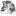# Fields Table

Below is a table with some fields that are in searches that will look for Bees Epifamily Anthophila (taxon_id=630955) that were recorded as being associated with the various Plant taxon as listed in the table below using the URL search:
``` https://www.inaturalist.org/observations?place_id=any&subview=map&taxon_id=Fauna Taxon ID Number of Choice&verifiable=any&field:Field of Choice=Flora Taxon of Choice Number) for instance: https://www.inaturalist.org/observations?place_id=any&subview=map&taxon_id=630955&verifiable=any&field:Interaction-%3EVisited%20flower%20of=48561 ```
One could look just for Bombus by substituting in the Taxon ID Number of 52775 eg https://www.inaturalist.org/observations?place_id=any&subview=map&taxon_id=52775&verifiable=any&field:Interaction-%3EVisited%20flower%20of=48561

Current numbers of bees as of today's date posting are in brackets eg =48561 (245)

Field Solidago (Goldenrods)=48678 Cirsium (Thistles)=48561 Grindelia (Gumplants)=55365 Grindelia stricta (Oregon Gumplant)=61992 Tetradymia canescens (Spineless Horsebrush)=79331
field:Interaction->Visited flower of= =48678 (1,028) =48561 (245) =55365 (25) =61992 (7) =79331 (0)
field:feeding on= =48678 (20) =48561 (12) =55365 (2) =61992 (1) =79331 (0)
field:Plant association= =48678 (30) =48561 (22) =55365 (149) =61992 (23) =79331 (1)
field:nectar / pollen delivering plant= =48678 (608) =48561 (452) =55365 (486) =61992 (61) =79331 (0)
field:name of associated plant= =48678 (343) =48561 (28) =55365 (10) =61992 (0) =79331 (0)
field:nectar plant= =48678 (203) =48561 (125) =55365 (74) =61992 (10) =79331 (1)
field:host plant id= =48678 (140) =48561 (3) =55365 (0) =61992 (0) =79331 (0)
field:host plant= =48678 (7) =48561 (3) =55365 (0) =61992 (1) =79331 (0)
field:Flower/Plant Name (what plant was the pollinator visiting?)= =48678 (0) =48561 (0) =55365 (0) =61992 (0) =79331 (0)

For the last Field the datatype was not based on taxon but instead text was selected which results in the taxon number not yielding a result.

Posted bybobmcd, November 26, 2022 06:30 AM